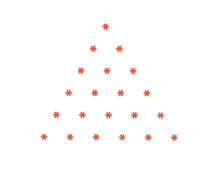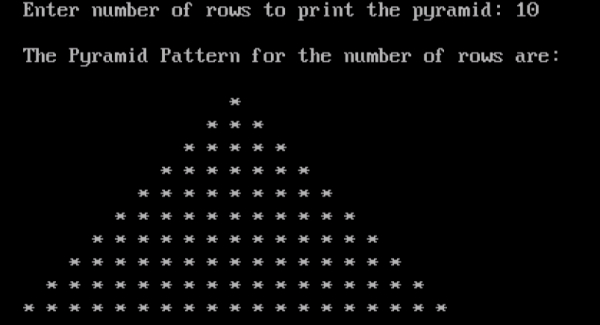# Program to print pyramid pattern in C

## Program Description

A pyramid is a polyhedron formed by connecting a polygonal base and a point, called the apex. Each base edge and apex form a triangle, called a lateral face. It is a conic solid with polygonal base. A pyramid with an n-sided base has n + 1 vertices, n + 1 faces, and 2n edges. All pyramids are self-dual.## Algorithm

Accept the number of rows from the user to form pyramid shape
Iterate the loop till the number of rows specified by the user:
Display 1 star in the first row
Increase the number of stars based on the number of rows.

## Example

/*Program to print Pyramid Pattern*/
#include<stdio.h>
int main() {
int r, s, rows=0;
int t=0;
clrscr();
printf("Enter number of rows to print the pyramid: ");
scanf("%d", &rows);
printf("");
printf("The Pyramid Pattern for the number of rows are:");
printf("");
for(r=1;r<=rows;++r,t=0) {
for(s=1; s<=rows-r; ++s){
printf(" ");
}
while (t!=2*r-1) {
printf("* ");
++t;
}
printf("");
}
getch();
return 0;
}

## Output